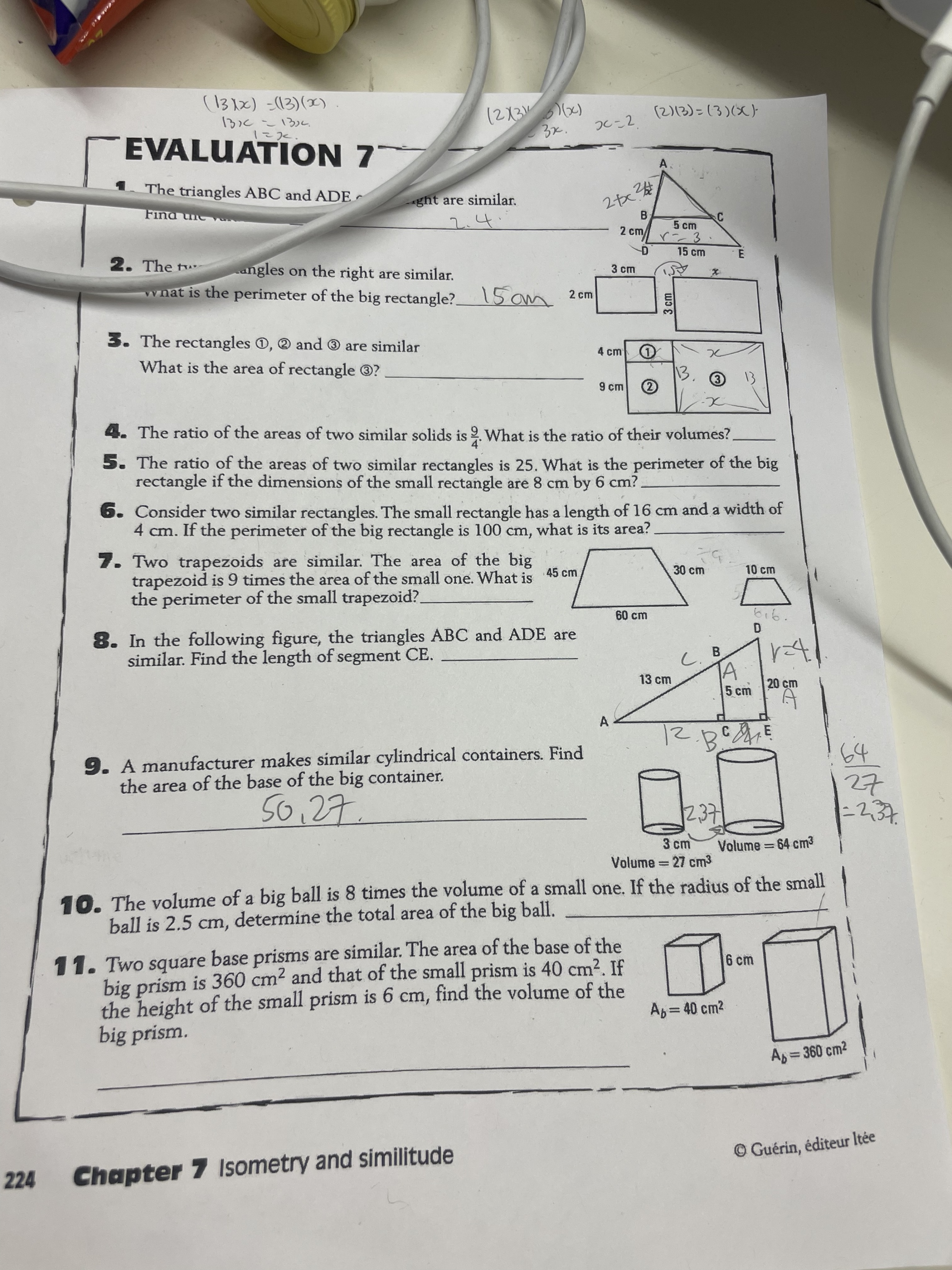## When Helping Means Winning contest

Ask a school-related question in the Help Zone and you could win a \$1000 scholarship!

# Help Zone

### Student QuestionMathematics## Explanations (1)

•Explanation from AlloprofExplanation from Alloprof

This Explanation was submitted by a member of the Alloprof team.

Hello there !

Regarding number 3, suppose the entirety of the length of the big rectangle, including 1,2 and3, being $$x$$. Use $$k$$ to find the ratio of the lengths, and then calculate the length of each rectangle with x. Then, equalize des areas and isolate for $$x$$.

Regarding number 8, find the measurement of $$AC$$ with trigonometric identities to afterwards use the $$k$$ ratio to find the sides of the triangle. Or, you could directly calculate the area and use $$k^{2}$$.

Thank you for using our services, and feel free to do so in the future !